# timeoptions

Create list of time plot options

## Description

Use the `timeoptions` command to create a `TimeOptions` object to customize time plot appearance. You can also use the command to override the plot preference settings in the MATLAB® session in which you create the time plots.

## Creation

### Syntax

``plotoptions = timeoptions``
``plotoptions = timeoptions('cstprefs')``

### Description

example

````plotoptions = timeoptions` returns a list of available options for time plots with default values set. You can use these options to customize the time plot appearance from the command line. ```

example

````plotoptions = timeoptions('cstprefs')` initializes the plot options with options you selected in the Control System Toolbox™ and System Identification Toolbox™ Preferences Editor. For more information about the editor, see Toolbox Preferences Editor. This syntax is useful when you want to change a few plot options but otherwise use your default preferences. A script that uses this syntax may generate results that look different when run in a session with different preferences.```

## Properties

expand all

Toggle response normalization, specified as either '`on`' or '`off`'.

Settling time threshold, specified as a positive scalar between values `0` and `1`.

Rise time limits between the values of `0` and `1`, specified as a two-element vector of the form `[min,max]`.

Time units, specified as one of the following values:

• `'nanoseconds'`

• `'microseconds'`

• `'milliseconds'`

• `'seconds'`

• `'minutes'`

• `'hours'`

• `'days'`

• `'weeks'`

• `'months'`

• `'years'`

You can also specify `'auto'` which uses time units specified in the `TimeUnit` property of the input system. For multiple systems with different time units, the units of the first system is used.

Number of standard deviations to use to plot the confidence region, specified as a scalar. This is applicable to identified models only.

Grouping of input-output (I/O) pairs, specified as one of the following:

• '`none`' — No input-output grouping.

• '`inputs`' — Group only the inputs.

• '`outputs`' — Group only the outputs.

• '`all`' — Group all the I/O pairs.

Input label style, specified as a structure with the following fields:

• `FontSize` — Font size, specified as a scalar value greater than zero in point units. The default font size depends on the specific operating system and locale. One point equals `1/72` inch.

• `FontWeight` — Character thickness, specified as '`Normal`' or '`bold`'. MATLAB uses the `FontWeight` property to select a font from those available on your system. Not all fonts have a bold weight. Therefore, specifying a bold font weight can still result in the normal font weight.

• `FontAngle` — Character slant, specified as '`Normal`' or '`italic`'. Not all fonts have both font styles. Therefore, the italic font might look the same as the normal font.

• `Color` — Text color, specified as an RGB triplet. The default color is dark grey with the RGB triplet `[0.4,0.4,0.4]`.

• `Interpreter` — Text interpreter, specified as one of these values:

• '`tex`' — Interpret characters using a subset of TeX markup. This is the default value of `Interpreter`.

• '`latex`' — Interpret characters using LaTeX markup.

• '`none`' — Display literal characters.

Output label style, specified as a structure with the following fields:

• `FontSize` — Font size, specified as a scalar value greater than zero in point units. The default font size depends on the specific operating system and locale. One point equals `1/72` inch.

• `FontWeight` — Character thickness, specified as '`Normal`' or '`bold`'. MATLAB uses the `FontWeight` property to select a font from those available on your system. Not all fonts have a bold weight. Therefore, specifying a bold font weight can still result in the normal font weight.

• `FontAngle` — Character slant, specified as '`Normal`' or '`italic`'. Not all fonts have both font styles. Therefore, the italic font might look the same as the normal font.

• `Color` — Text color, specified as an RGB triplet. The default color is dark grey with the RGB triplet `[0.4,0.4,0.4]`.

• `Interpreter` — Text interpreter, specified as one of these values:

• '`tex`' — Interpret characters using a subset of TeX markup. This is the default value of `Interpreter`.

• '`latex`' — Interpret characters using LaTeX markup.

• '`none`' — Display literal characters.

Toggle display of inputs, specified as either `{'on'}` or `{'off'}`.

Toggle display of outputs, specified as either `{'on'}` or `{'off'}`.

Title text and style, specified as a structure with the following fields:

• `String` — Label text, specified as a character vector. By default, the plot is titled 'Time Response'.

• `FontSize` — Font size, specified as a scalar value greater than zero in point units. The default font size depends on the specific operating system and locale. One point equals `1/72` inch.

• `FontWeight` — Character thickness, specified as '`Normal`' or '`bold`'. MATLAB uses the `FontWeight` property to select a font from those available on your system. Not all fonts have a bold weight. Therefore, specifying a bold font weight can still result in the normal font weight.

• `FontAngle` — Character slant, specified as '`Normal`' or '`italic`'. Not all fonts have both font styles. Therefore, the italic font might look the same as the normal font.

• `Color` — Text color, specified as an RGB triplet. The default color is black specified by the RGB triplet `[0,0,0]`.

• `Interpreter` — Text interpreter, specified as one of these values:

• '`tex`' — Interpret characters using a subset of TeX markup. This is the default value of `Interpreter`.

• '`latex`' — Interpret characters using LaTeX markup.

• '`none`' — Display literal characters.

X-axis label text and style, specified as a structure with the following fields:

• `String` — Label text, specified as a character vector. By default, the axis is titled 'Time'.

• `FontSize` — Font size, specified as a scalar value greater than zero in point units. The default font size depends on the specific operating system and locale. One point equals `1/72` inch.

• `FontWeight` — Character thickness, specified as '`Normal`' or '`bold`'. MATLAB uses the `FontWeight` property to select a font from those available on your system. Not all fonts have a bold weight. Therefore, specifying a bold font weight can still result in the normal font weight.

• `FontAngle` — Character slant, specified as '`Normal`' or '`italic`'. Not all fonts have both font styles. Therefore, the italic font might look the same as the normal font.

• `Color` — Text color, specified as an RGB triplet. The default color is black specified by the RGB triplet `[0,0,0]`.

• `Interpreter` — Text interpreter, specified as one of these values:

• '`tex`' — Interpret characters using a subset of TeX markup. This is the default value of `Interpreter`.

• '`latex`' — Interpret characters using LaTeX markup.

• '`none`' — Display literal characters.

Y-axis label text and style, specified as a structure with the following fields:

• `String` — Label text, specified as a cell array of character vectors. By default, the axis is titled 'Amplitude'.

• `FontSize` — Font size, specified as a scalar value greater than zero in point units. The default font size depends on the specific operating system and locale. One point equals `1/72` inch.

• `FontWeight` — Character thickness, specified as '`Normal`' or '`bold`'. MATLAB uses the `FontWeight` property to select a font from those available on your system. Not all fonts have a bold weight. Therefore, specifying a bold font weight can still result in the normal font weight.

• `FontAngle` — Character slant, specified as '`Normal`' or '`italic`'. Not all fonts have both font styles. Therefore, the italic font might look the same as the normal font.

• `Color` — Text color, specified as an RGB triplet. The default color is black specified by the RGB triplet `[0,0,0]`.

• `Interpreter` — Text interpreter, specified as one of these values:

• '`tex`' — Interpret characters using a subset of TeX markup. This is the default value of `Interpreter`.

• '`latex`' — Interpret characters using LaTeX markup.

• '`none`' — Display literal characters.

Tick label style, specified as a structure with the following fields:

• `FontSize` — Font size, specified as a scalar value greater than zero in point units. The default font size depends on the specific operating system and locale. One point equals `1/72` inch.

• `FontWeight` — Character thickness, specified as '`Normal`' or '`bold`'. MATLAB uses the `FontWeight` property to select a font from those available on your system. Not all fonts have a bold weight. Therefore, specifying a bold font weight can still result in the normal font weight.

• `FontAngle` — Character slant, specified as '`Normal`' or '`italic`'. Not all fonts have both font styles. Therefore, the italic font might look the same as the normal font.

• `Color` — Text color, specified as an RGB triplet. The default color is black specified by the RGB triplet `[0,0,0]`.

Toggle grid display on the plot, specified as either '`off`' or '`on`'.

Color of the grid lines, specified as an RGB triplet. The default color is light grey specified by the RGB triplet `[0.15,0.15,0.15]`.

Selection mode for the x-axis limits, specified as one of these values:

• '`auto`' — Enable automatic limit selection, which is based on the total span of the plotted data.

• '`manual`' — Manually specify the axis limits. To specify the axis limits, set the `XLim` property.

Selection mode for the y-axis limits, specified as one of these values:

• '`auto`' — Enable automatic limit selection, which is based on the total span of the plotted data.

• '`manual`' — Manually specify the axis limits. To specify the axis limits, set the `YLim` property.

X-axis limits, specified as a cell array of two-element vector of the form `[min,max]`.

Y-axis limits, specified as a cell array of two-element vector of the form `[min,max]`.

## Object Functions

 `getoptions` Return plot options handle or plot options property `impulseplot` Plot impulse response with additional plot customization options `initialplot` (Control System Toolbox) Plot initial condition response with additional plot customization options `lsimplot` Plot simulated time response of dynamic system to arbitrary inputs with additional plot customization options `setoptions` Set plot options handle or plot options property `stepplot` Plot step response with additional plot customization options

## Examples

collapse all

Create a default time options set.

`opt = timeoptions;`

Enable plotting of normalized responses.

`opt.Normalize = 'on';`

Plot the step response of two transfer function models using the specified options.

```sys1 = tf(10,[1,1]); sys2 = tf(5,[1,5]); stepplot(sys1,sys2,opt);```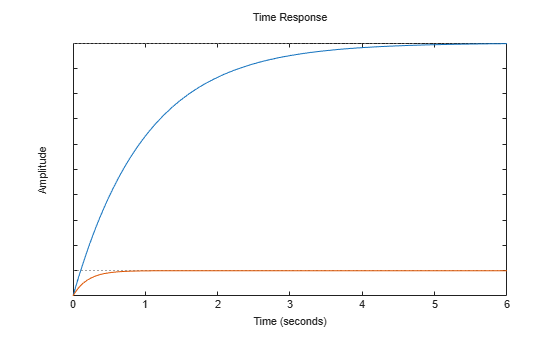The plot shows the normalized step response for the two transfer function models.

For this example, use the plot handle to change the time units to minutes and turn on the grid.

Generate a random state-space model with 5 states and create the step response plot with plot handle `h`.

```rng("default") sys = rss(5); h = stepplot(sys);```

Change the time units to minutes and turn on the grid. To do so, edit properties of the plot handle, `h` using `setoptions`.

`setoptions(h,'TimeUnits','minutes','Grid','on');`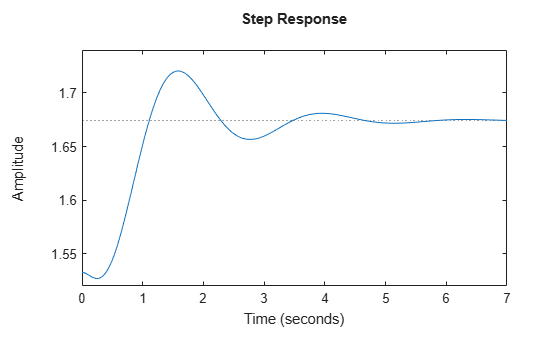The step plot automatically updates when you call `setoptions`.

Alternatively, you can also use the `timeoptions` command to specify the required plot options. First, create an options set based on the toolbox preferences.

`plotoptions = timeoptions('cstprefs');`

Change properties of the options set by setting the time units to minutes and enabling the grid.

```plotoptions.TimeUnits = 'minutes'; plotoptions.Grid = 'on'; stepplot(sys,plotoptions);```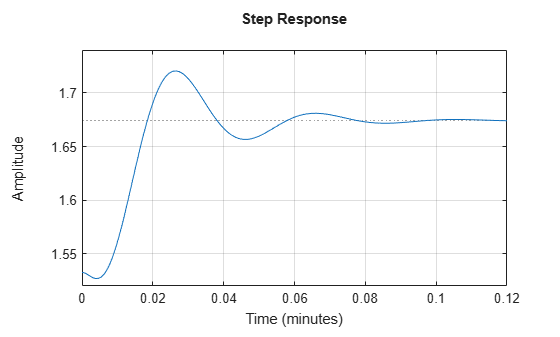You can use the same option set to create multiple step plots with the same customization. Depending on your own toolbox preferences, the plot you obtain might look different from this plot. Only the properties that you set explicitly, in this example `TimeUnits` and `Grid`, override the toolbox preferences.

For this example, examine the step response of the following zero-pole-gain model and limit the step plot to `tFinal` = 15 s. Use 15-point blue text for the title. This plot should look the same, regardless of the preferences of the MATLAB session in which it is generated.

```sys = zpk(-1,[-0.2+3j,-0.2-3j],1)*tf([1 1],[1 0.05]); tFinal = 15;```

First, create a default options set using `timeoptions`.

`plotoptions = timeoptions;`

Next change the required properties of the options set `plotoptions`.

```plotoptions.Title.FontSize = 15; plotoptions.Title.Color = [0 0 1];```

Now, create the step response plot using the options set `plotoptions`.

`h = stepplot(sys,tFinal,plotoptions);`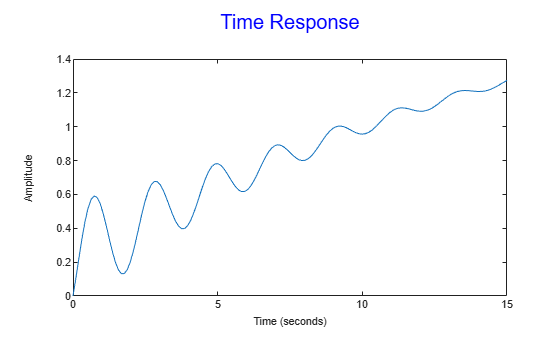Because `plotoptions` begins with a fixed set of options, the plot result is independent of the toolbox preferences of the MATLAB session.

By default, `lsimplot` simulates the model assuming all states are zero at the start of the simulation. When simulating the response of a state-space model, use the optional `x0` input argument to specify nonzero initial state values. Consider the following two-state SISO state-space model.

```A = [-1.5 -3; 3 -1]; B = [1.3; 0]; C = [1.15 2.3]; D = 0; sys = ss(A,B,C,D);```

Suppose that you want to allow the system to evolve from a known set of initial states with no input for 2 s, and then apply a unit step change. Specify the vector `x0` of initial state values, and create the input vector.

```x0 = [-0.2 0.3]; t = 0:0.05:8; u = zeros(length(t),1); u(t>=2) = 1;```

First, create a default options set using `timeoptions`.

`plotoptions = timeoptions;`

Next change the required properties of the options set `plotoptions` and plot the simulated response with the zero order hold option.

```plotoptions.Title.FontSize = 15; plotoptions.Title.Color = [0 0 1]; plotoptions.Grid = 'on'; h = lsimplot(sys,u,t,x0,plotoptions,'zoh'); hold on title('Simulated Time Response with Initial Conditions')```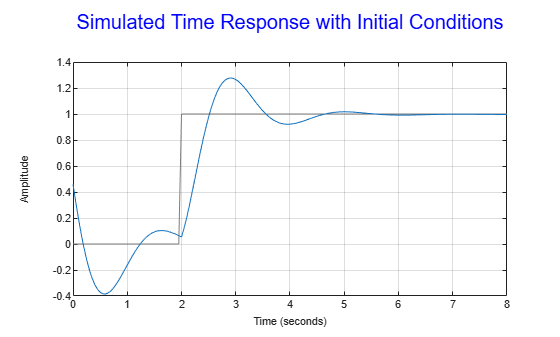The first half of the plot shows the free evolution of the system from the initial state values `[-0.2 0.3]`. At `t = 2` there is a step change to the input, and the plot shows the system response to this new signal beginning from the state values at that time. Because `plotoptions` begins with a fixed set of options, the plot result is independent of the toolbox preferences of the MATLAB session.

For this example, change time units to minutes and turn the grid on for the simulated response plot. Consider the following transfer function.

`sys = tf(3,[1 2 3]);`

To compute the response of this system to an arbitrary input signal, provide `lsimplot` with a vector of the times `t` at which you want to compute the response and a vector `u` containing the corresponding signal values. For instance, plot the system response to a ramping step signal that starts at 0 at time `t = 0`, ramps from 0 at `t = 1` to 1 at `t = 2`, and then holds steady at 1. Define `t` and compute the values of `u`.

```t = 0:0.04:8; u = max(0,min(t-1,1));```

Use `lsimplot` plot the system response to the signal with a plot handle `h`.

`h = lsimplot(sys,u,t);`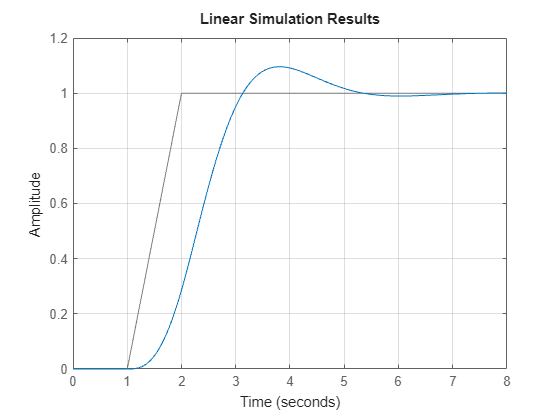The plot shows the applied input `(u,t)` in gray and the system response in blue.

Use the plot handle to change the time units to minutes and to turn the grid on. To do so, edit properties of the plot handle, `h` using `setoptions`.

`setoptions(h,'TimeUnits','minutes','Grid','on')`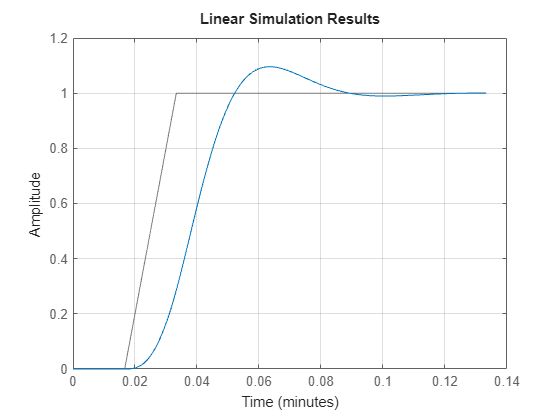The plot automatically updates when you call `setoptions`.

Alternatively, you can also use the `timeoptions` command to specify the required plot options. First, create an options set based on the toolbox preferences.

`plotoptions = timeoptions('cstprefs');`

Change properties of the options set by setting the time units to minutes and enabling the grid.

```plotoptions.TimeUnits = 'minutes'; plotoptions.Grid = 'on'; lsimplot(sys,u,t,plotoptions);```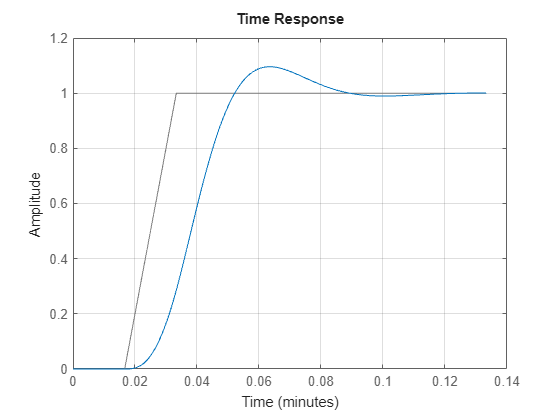## Version History

Introduced in R2012a